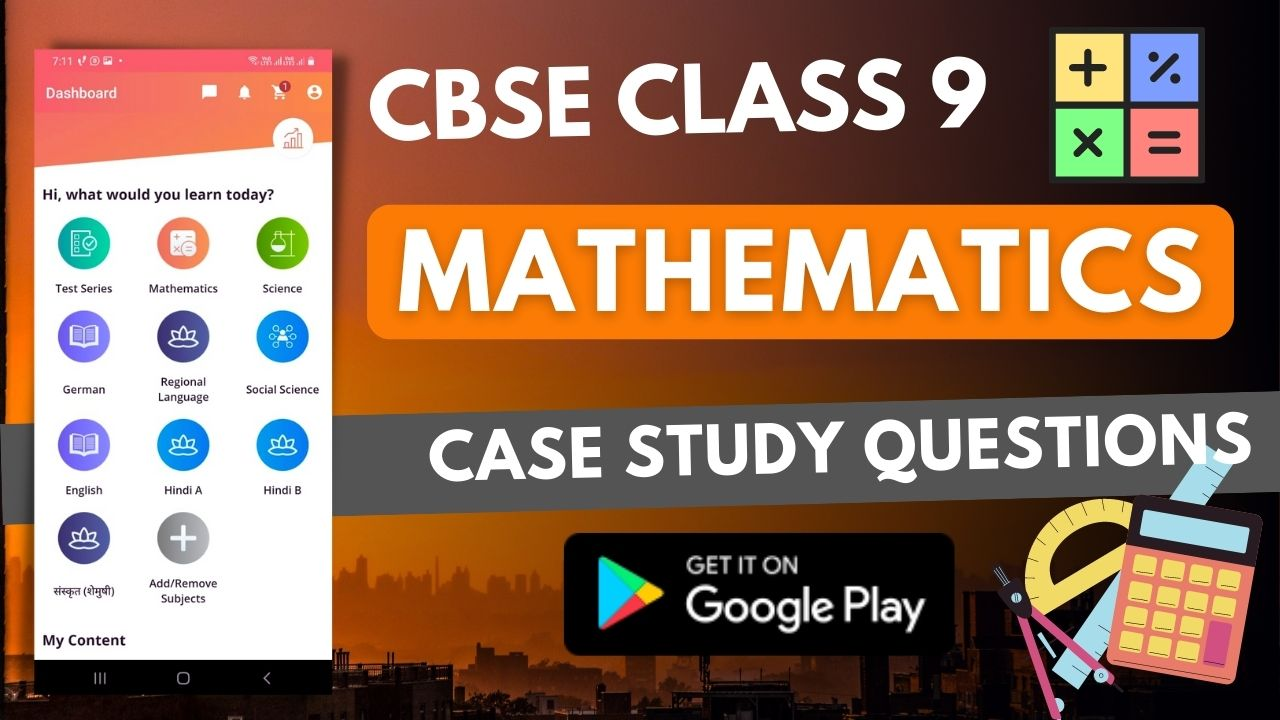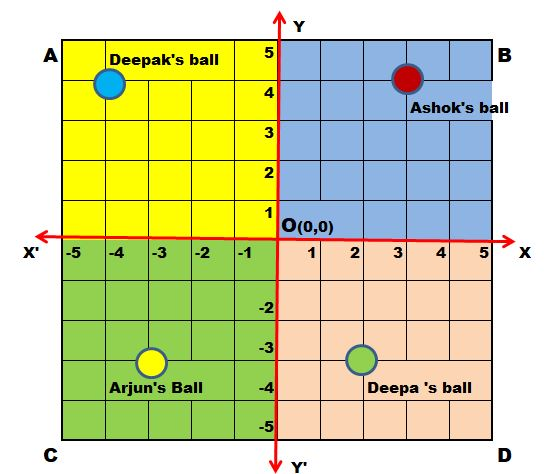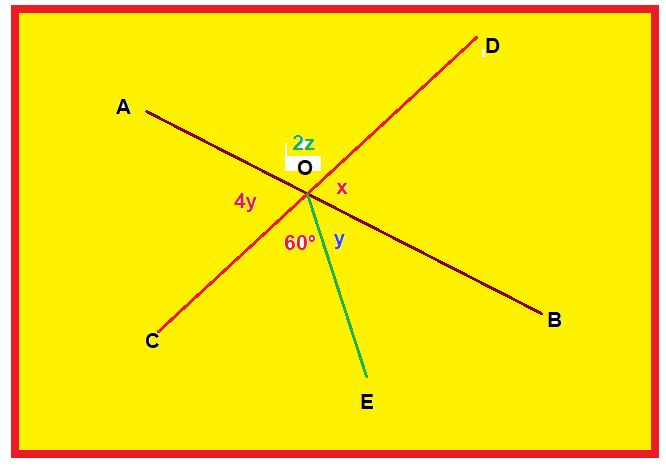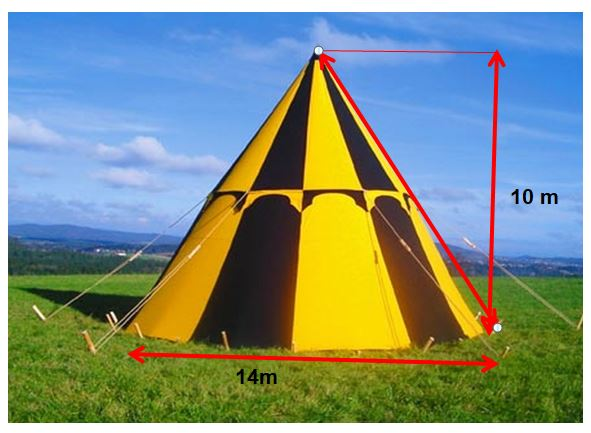1. /
2. CBSE
3. /
4. Class 09
5. /
6. Mathematics
7. /
8. CBSE Class 9 Mathematics...

# CBSE Class 9 Mathematics Case Study Questions### myCBSEguide App

Download the app to get CBSE Sample Papers 2023-24, NCERT Solutions (Revised), Most Important Questions, Previous Year Question Bank, Mock Tests, and Detailed Notes.

If you’re looking for a comprehensive and reliable study resource and case study questions for class 9 CBSE, myCBSEguide is the perfect door to enter. With over 10,000 study notes, solved sample papers and practice questions, it’s got everything you need to ace your exams. Plus, it’s updated regularly to keep you aligned with the latest CBSE syllabus. So why wait? Start your journey to success with myCBSEguide today!

## Significance of Mathematics in Class 9

Mathematics is an important subject for students of all ages. It helps students to develop problem-solving and critical-thinking skills, and to think logically and creatively. In addition, mathematics is essential for understanding and using many other subjects, such as science, engineering, and finance.

CBSE Class 9 is an important year for students, as it is the foundation year for the Class 10 board exams. In Class 9, students learn many important concepts in mathematics that will help them to succeed in their board exams and in their future studies. Therefore, it is essential for students to understand and master the concepts taught in Class 9 Mathematics.## Case studies in Class 9 Mathematics

A case study in mathematics is a detailed analysis of a particular mathematical problem or situation. Case studies are often used to examine the relationship between theory and practice, and to explore the connections between different areas of mathematics. Often, a case study will focus on a single problem or situation and will use a variety of methods to examine it. These methods may include algebraic, geometric, and/or statistical analysis.

## Example of Case study questions in Class 9 Mathematics

The Central Board of Secondary Education (CBSE) has included case study questions in the Class 9 Mathematics paper. This means that Class 9 Mathematics students will have to solve questions based on real-life scenarios. This is a departure from the usual theoretical questions that are asked in Class 9 Mathematics exams.

The following are some examples of case study questions from Class 9 Mathematics:

### Class 9 Mathematics Case study question 1There is a square park ABCD in the middle of Saket colony in Delhi. Four children Deepak, Ashok, Arjun and Deepa went to play with their balls. The colour of the ball of Ashok, Deepak,  Arjun and Deepa are red, blue, yellow and green respectively.
All four children roll their ball from centre point O in the direction of  XOY, X’OY, X’OY’ and XOY’. Their balls stopped as shown in the above image.

1. What are the coordinates of the ball of Ashok?
1. (4, 3)
2. (3, 4)
3. (4, 4)
4. (3, 3)
2. What are the coordinates of the ball of Deepa?
1. (2, -3)
2. (3, 2)
3. (2, 3)
4. (2, 2)
3. What the line XOX’ is called?
1. y-axis
2. ordinate
3. x-axis
4. origin
4. What the point O (0,0) is called?
1. y-axis
2. ordinate
3. x-axis
4. origin
5. What is the ordinate of the ball of Arjun?
1. -3
2. 3
3. 4
4. 2

1. (b) (3,4)
2. (a) (2,-3)
3. (c) x-axis
4. (d) Origin
5. (a) -3

### Class 9 Mathematics Case study question 2

Maths teacher draws a straight line AB shown on the blackboard as per the following figure.1. Now he told Raju to draw another line CD as in the figure
2. The teacher told Ajay to mark AOD  as 2z
3. Suraj was told to mark AOC as 4y
4. Clive Made and angle COE = 60°
5. Peter marked BOE and BOD as y and x respectively

1. What is the value of x?
1. 48°
2. 96°
3. 100°
4. 120°
2. What is the value of y?
1. 48°
2. 96°
3. 100°
4. 24°
3. What is the value of z?
1. 48°
2. 96°
3. 42°
4. 120°
4. What should be the value of x + 2z?
1. 148°
2. 360°
3. 180°
4. 120°
5. What is the relation between y and z?
1. 2y + z = 90°
2. 2y + z = 180°
3. 4y + 2z = 120°
4. y = 2z

1. (b) 96°
2. (d) 24°
3. (c) 42°
4. (c) 180°
5. (a) 2y + z = 90°

### Class 9 Mathematics Case study question 3

Once four friends Rahul, Arun, Ajay and Vijay went for a picnic at a hill station. Due to peak season, they did not get a proper hotel in the city. The weather was fine so they decided to make a conical tent at a park. They were carrying 300 m² cloth with them. As shown in the figure they made the tent with height 10 m and diameter 14 m. The remaining cloth was used for the floor.1. How much Cloth was used for the floor?
1. 31.6 m²
2. 16 m²
3. 10 m²
4. 20 m²
2. What was the volume of the tent?
1. 300 m³
2. 160 m³
3. 513.3 m³
4. 500 m³
3. What was the area of the floor?
1. 50 m²
2. 100 m²
3. 150 m²
4. 154 m²
4. What was the total surface area of the tent?
1. 400 m²
2. 422.4 m²
3. 300 m²
4. 400 m²
5. What was the latent height of the tent?
1. 12 m
2. 12.2 m
3. 15 m
4. 17 m

1. (a) 31.6 m²
2. (c) 513.3 m³
3. (d) 154 m²
4. (b) 422.4 m²
5. (b) 12.2 m

Class 9 Mathematics Case study question 4

The weights of newborn babies (in kg.) are recorded to maintain the health report cards in Apollo hospital on regular basis. On a particular day the weights of newborn recorded are are as follows:
3.1, 3.0, 2.9, 2.9, 2.8, 2.8, 2.7, 2.7, 2.6, 2.5, 2.5, 2.4, 2.3, 2.2, 2.1.1. Determine the range.
1. 1.0 Kg
2. 2.1 Kg
3. 2.7 Kg
4. 2.0 kg
2. How many babies were born on that day?
1. 11
2. 15
3. 12
4. 16
3. How many babies weigh below 2.5 kg?
1. 3
2. 4
3. 5
4. 6
4. How many babies weigh more than 2.8 kg?
1. 1
2. 2
3. 3
4. 4
5. How many babies weigh 2.8 kg
1. 1
2. 2
3. 3
4. 4

1. (a) 1.0 Kg
2. (b) 15
3. (c) 4
4. (d) 4
5. (b) 2

How to Answer Class 9 Mathematics Case study questions

To crack case study questions, Class 9 Mathematics students need to apply their mathematical knowledge to real-life situations. They should first read the question carefully and identify the key information. They should then identify the relevant mathematical concepts that can be applied to solve the question. Once they have done this, they can start solving the Class 9 Mathematics case study question.

Students need to be careful while solving the Class 9 Mathematics case study questions. They should not make any assumptions and should always check their answers. If they are stuck on a question, they should take a break and come back to it later. With some practice, the Class 9 Mathematics students will be able to crack case study questions with ease.

## Class 9 Mathematics Curriculum at Glance

At the secondary level, the curriculum focuses on improving students’ ability to use Mathematics to solve real-world problems and to study the subject as a separate discipline. Students are expected to learn how to solve issues using algebraic approaches and how to apply their understanding of simple trigonometry to height and distance problems. Experimenting with numbers and geometric forms, making hypotheses, and validating them with more observations are all part of Math learning at this level.

The suggested curriculum covers number systems, algebra, geometry, trigonometry, mensuration, statistics, graphing, and coordinate geometry, among other topics. Math should be taught through activities that include the use of concrete materials, models, patterns, charts, photographs, posters, and other visual aids.

CBSE Class 9 Mathematics (Code No. 041)

 Units Unit Name Marks I NUMBER SYSTEMS 10 II ALGEBRA 20 III COORDINATE GEOMETRY 04 IV GEOMETRY 27 V MENSURATION 13 VI STATISTICS & PROBABILITY 06 Total 80

## Class 9 Mathematics question paper design

The CBSE Class 9 mathematics question paper design is intended to measure students’ grasp of the subject’s fundamental ideas. The paper will put their problem-solving and analytical skills to the test. Class 9 mathematics students are advised to go through the question paper pattern thoroughly before they start preparing for their examinations. This will help them understand the paper better and enable them to score maximum marks. Refer to the given Class 9 Mathematics question paper design.

### QUESTION PAPER DESIGN (CLASS 9 MATHEMATICS)

 S.No Typology of Questions Total Marks % Weightage (approx) 1. Remembering: Exhibit memory of previously learned material by recalling facts, terms, basic concepts, and answers. Understanding: Demonstrate understanding of facts and ideas by organizing, comparing, translating, interpreting, giving descriptions, and stating main ideas 43 54 2. Applying: Solve problems to new situations by applying acquired knowledge, facts, techniques and rules in a different way. 19 24 3. Analysing: Examine and break information into parts by identifying motives or causes. Make inferences and find evidence to support generalizations Evaluating: Present and defend opinions by making judgments about information, validity of ideas, or quality of work based on a set of criteria. Creating: Compile information together in a different way by combining elements in a new pattern or proposing alternative solutions 18 22 Total 80 100

## myCBSEguide: Blessing in disguise

Class 9 is an important milestone in a student’s life. It is the last year of high school and the last chance to score well in the CBSE board exams. myCBSEguide is the perfect platform for students to get started on their preparations for Class 9 Mathematics. myCBSEguide provides comprehensive study material for all subjects, including practice questions, sample papers, case study questions and mock tests. It also offers tips and tricks on how to score well in exams. myCBSEguide is the perfect door to enter for class 9 CBSE preparations.### Test Generator

Create question paper PDF and online tests with your own name & logo in minutes.### myCBSEguide

Question Bank, Mock Tests, Exam Papers, NCERT Solutions, Sample Papers, Notes

### 6 thoughts on “CBSE Class 9 Mathematics Case Study Questions”

1. This method is not easy for me

2. aarti and rashika are two classmates. due to exams approaching in some days both decided to study together. during revision hour both find difficulties and they solved each other’s problems. aarti explains simplification of 2+ ?2 by rationalising the denominator and rashika explains 4+ ?2 simplification of (v10-?5)(v10+ ?5) by using the identity (a – b)(a+b). based on above information, answer the following questions: 1) what is the rationalising factor of the denominator of 2+ ?2 a) 2-?2 b) 2?2 c) 2+ ?2 by rationalising the denominator of aarti got the answer d) a) 4+3?2 b) 3+?2 c) 3-?2 4+ ?2 2+ ?2 d) 2-?3 the identity applied to solve (?10-?5) (v10+ ?5) is a) (a+b)(a – b) = (a – b)² c) (a – b)(a+b) = a² – b² d) (a-b)(a+b)=2(a² + b²) ii) b) (a+b)(a – b) = (a + b

3. MATHS PAAGAL HAI

4. All questions was easy but search ? hard questions. These questions was not comparable with cbse. It was totally wastage of time.

5. i am happy

6. High